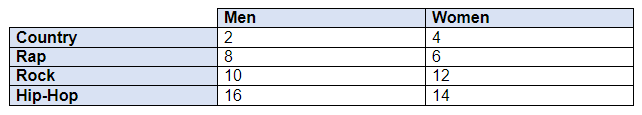## Q. When should I use a chi-square test?

Chi-square tests are used when you are comparing two categorical variables. You should perform a chi-square test when you are working with counts of records in different groups. This is different from other hypothesis tests (for example, a t-test) where you are comparing a numerical variable across different groups (i.e. across a categorical variable).

For example, if you were trying to compare the average number of minutes that men and women exercise each day, you would use a hypothesis test. You have a categorical variable (gender) with two levels (men and women) and a numerical quantity of interest (minutes exercised per day). But what if you wanted to compare favorite genre of music while exercising between men and women? This would be a situation for a chi-square test. There are two categorical variables, gender and music genre. Gender has two levels (men and women) and suppose genre has 4 levels (country, rap, rock, and hip-hop). Chi-square tests are often represented with contingency tables like the one shown below. Contingency tables show the observed counts for each group.### Topics

• Last Updated Apr 20, 2021
• Views 0